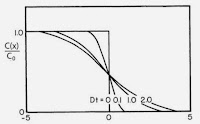## Friday, May 15, 2015

### What My Dogs Forced Me To Learn About Thermal Energy Transfer

I’m a dog lover, so I have to enjoy an American Journal of Physics paper that begins “For many years, I have competed and judged in American Kennel Club obedience trials.” The title of the paper is also delightful: “What my Dogs Forced Me to Learn About Thermal Energy Transfer” (Craig Bohren, American Journal of Physics, Volume 83, Pages 443−446, 2015). Bohren’s hypothesis is that an animal perceives hotness and coldness not directly from an object’s temperature, as one might naively expect, but from the flux density of thermal energy. I could follow his analysis of this idea, but I prefer to use the 5th edition of Intermediate Physics for Medicine and Biology, because Russ Hobbie and I have already worked out almost all the results we need.

Chapter 4 of IPMB analyzes diffusion. We consider the concentration, C, of particles as they diffuse in one dimension. Initially (t = 0), there exists a concentration difference C0 between the left and right sides of a boundary at x = 0. We solve the diffusion equation in this case, and find the concentration in terms of an error function

C(x,t) = C0/2 [ 1 – erf(x/√4Dt) ] ,                  Eq. 4.75

where D is the diffusion constant. A plot of C(x,t) is shown in Fig. 4.22 (we assume C = 0 on the far right, but you could add a constant to the solution without changing the physics, so all that really matters is the concentration difference).Fig. 4.22 The spread of an initially sharp boundary due to diffusion.
In Homework Problem 19, Russ and I show that the analysis of particle diffusion is equivalent to an analysis of heat conduction, with the thermal diffusivity D given by the thermal conductivity, κ, divided by the specific heat capacity, c, and the density, ρ

D = κ/cρ .

So, by analogy, if you start (t = 0) with a uniform temperature on the left and on the right sides of a boundary at x = 0, with an initial temperature difference ΔT between sides, the temperature distribution T(x,t) is (to within a additive constant temperature) the same as the concentration distribution calculated earlier.

T(x,t) = ΔT /2 [ 1 – erf(x/√4Dt) ] .

The temperature of the interface is always ΔT/2. If a dog responded to simply the temperature at x = 0 (where its thermoreceptors are presumably located), it would react in a way strictly proportional to the temperature difference ΔT. But Bohren’s hypothesis is that thermoreceptors respond to the energy flux density, κ dT/dx.

Now let us look again at Fig. 4.22. The slope of the curve at x = 0 is the key quantity. So, we must differentiate our expression for T(x,t). We get

κ dT/dx = - κ ΔT/2 d/dx [ erf(x/√4Dt) ] .

By the chain rule, this becomes (with u = x/√4Dt)

κ dT/dx  = - κ ΔT / (2 √4Dt ) d(erf(u))/du .

The derivative of the error function is given in IPMB on page 179

d/du (erf(u)) = 2/√π e-u2 .

At the interface (u = 0), this becomes 2/√π. Therefore

κ dT/dx = - κ ΔT /√4πDt .

Bohren comes to the same result, but by a slightly different argument.

The energy flux density depends on time, with an infinite response initially (we assume an abrupt difference of temperature on the two sides of the boundary x = 0) that falls to zero as the time becomes large. The flux density depends on the material parameters by the quantity κ/√D , which is equivalent to √cρκ and is often called the thermal inertia.

Bohren goes on to analyze the case when the two sides have different properties (for example, the left might be a piece of aluminum, and the right a dog’s tongue), and shows that you get a similar result except the effective thermal inertia is a combination of the thermal inertia on the left and right. He does not solve the entire bioheat equation (Sec. 14.11 in IPMB), including the effect of blood flow. I would guess that blood flow would have little effect initially, but it would play a greater and greater role as time goes by.

Perhaps I will try Bohren’s experiment: I’ll give Auggie (my daughter Kathy’s lovable foxhound) a cylinder of aluminum and a cylinder of stainless steel, and see if he can distinguish between the two. My prediction is that, rather than either metal, he prefers rawhide.